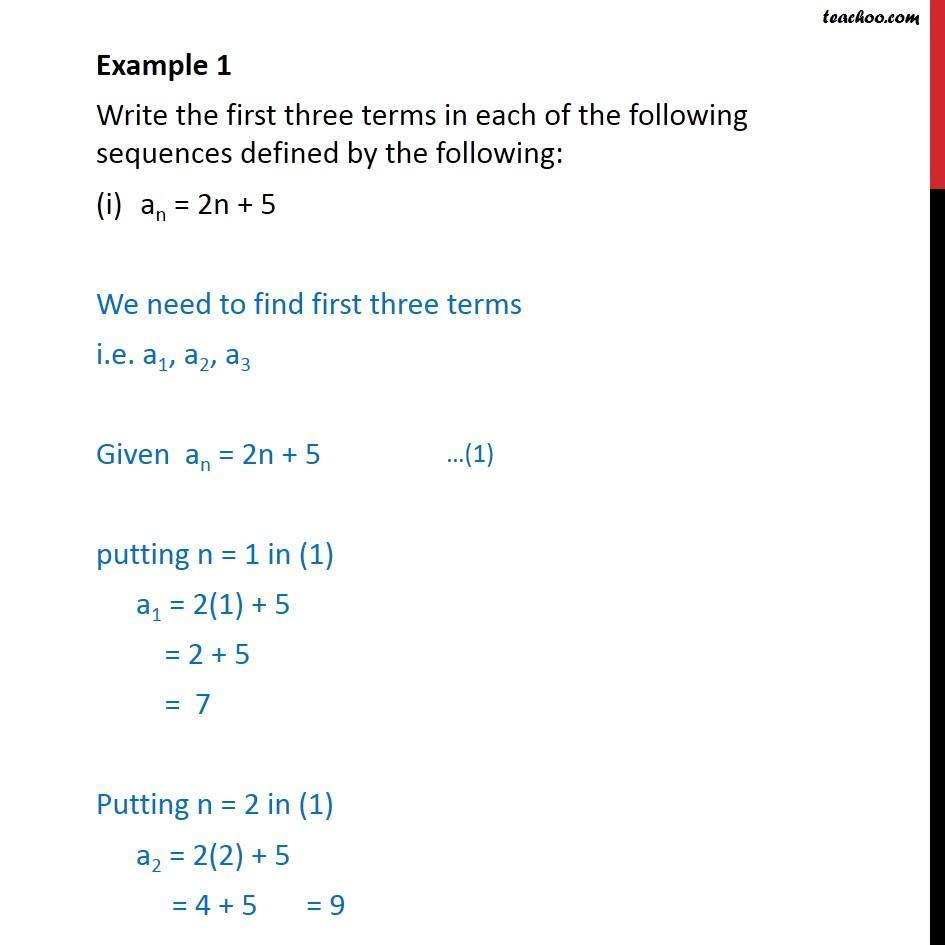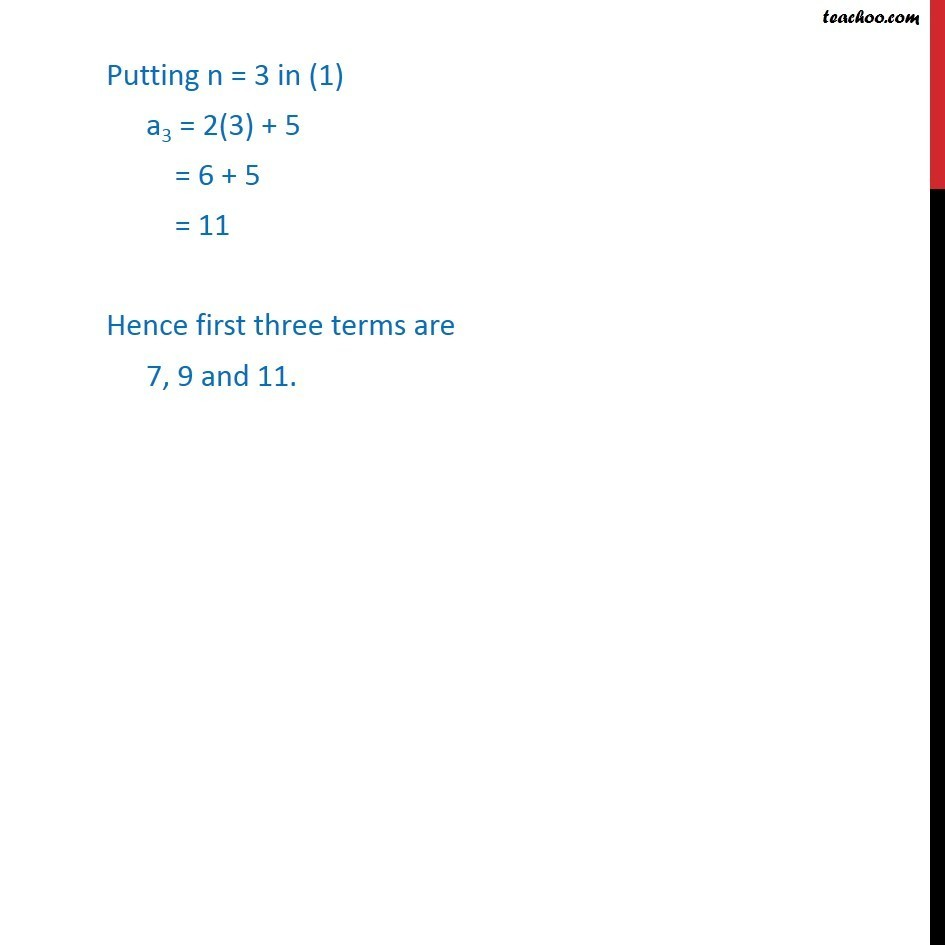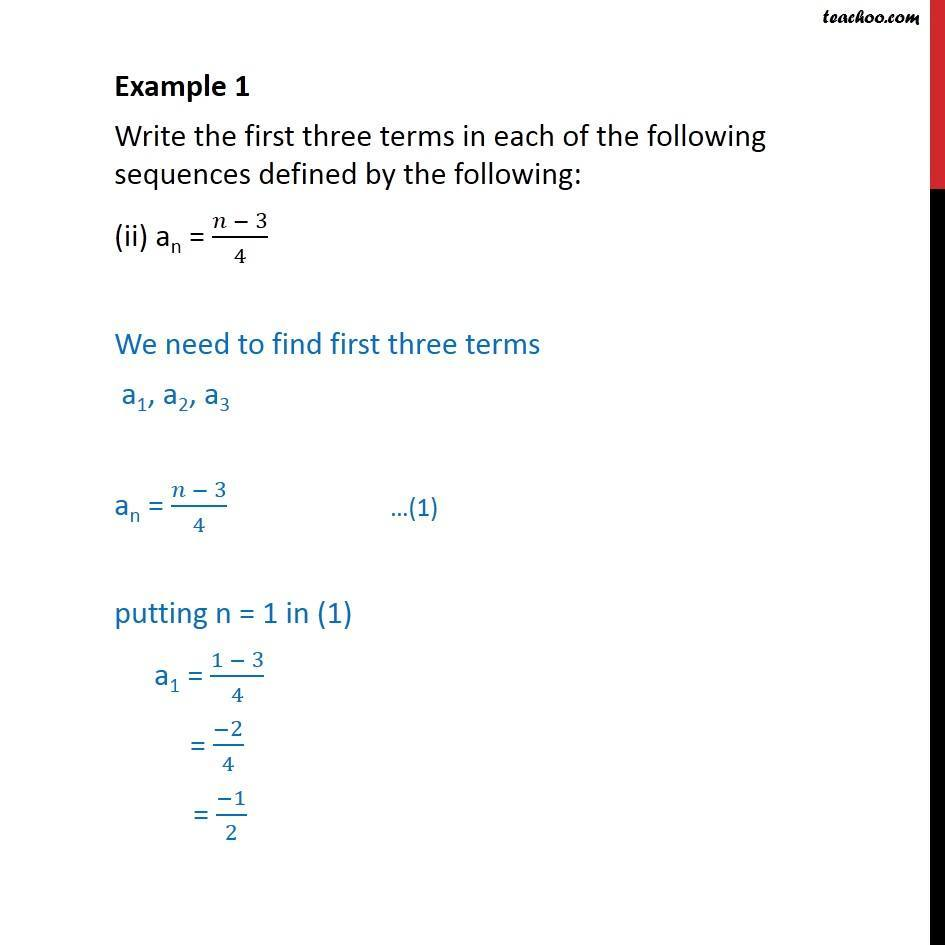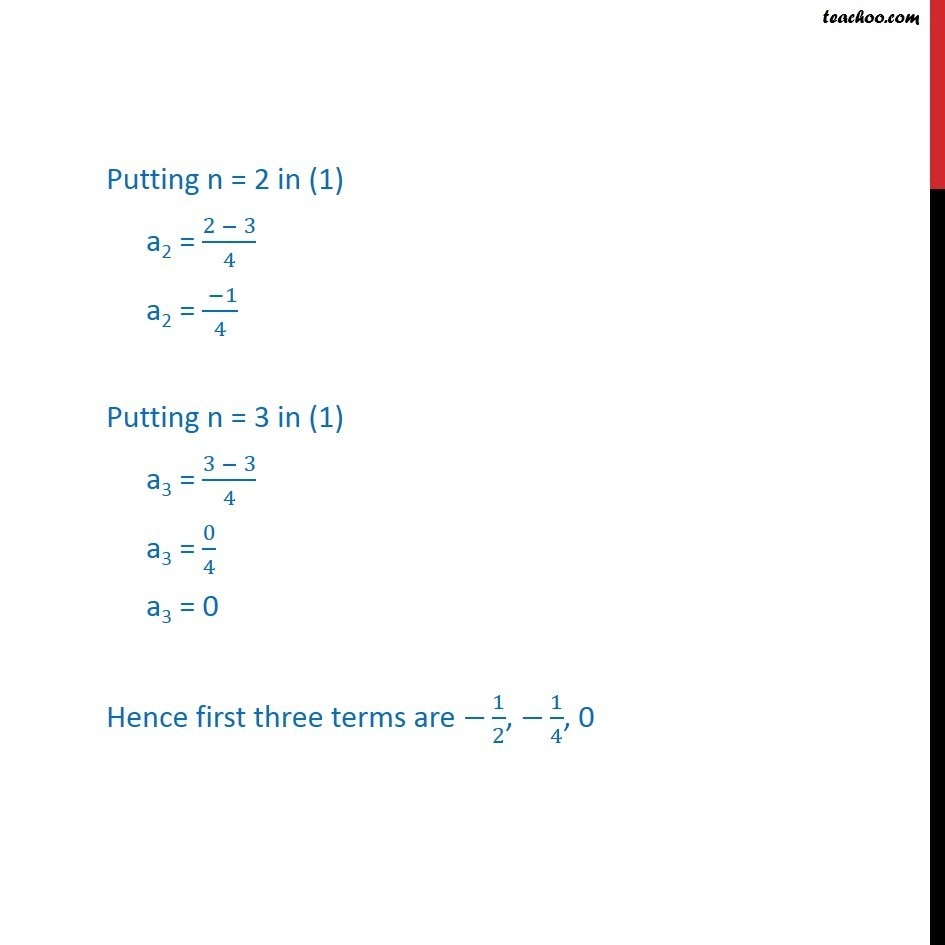Subscribe to our Youtube Channel - https://you.tube/teachoo

1. Chapter 9 Class 11 Sequences and Series
2. Concept wise
3. Finding Sequences

Transcript

Example 1 Write the first three terms in each of the following sequences defined by the following: an = 2n + 5 We need to find first three terms i.e. a1, a2, a3 Given an = 2n + 5 putting n = 1 in (1) a1 = 2(1) + 5 = 2 + 5 = 7 Putting n = 2 in (1) a2 = 2(2) + 5 = 4 + 5 Putting n = 3 in (1) a3 = 2(3) + 5 = 6 + 5 = 11 Hence first three terms are 7, 9 and 11. Example 1 Write the first three terms in each of the following sequences defined by the following: (ii) an = (𝑛 − 3)/4 We need to find first three terms a1, a2, a3 an = (𝑛 − 3)/4 putting n = 1 in (1) a1 = (1 − 3)/4 = (−2)/4 = (−1)/2 Putting n = 2 in (1) a2 = (2 − 3)/4 a2 = ( −1)/4 Putting n = 3 in (1) a3 = (3 − 3)/4 a3 = 0/4 a3 = 0 Hence first three terms are −1/2, −1/4, 0

Finding Sequences